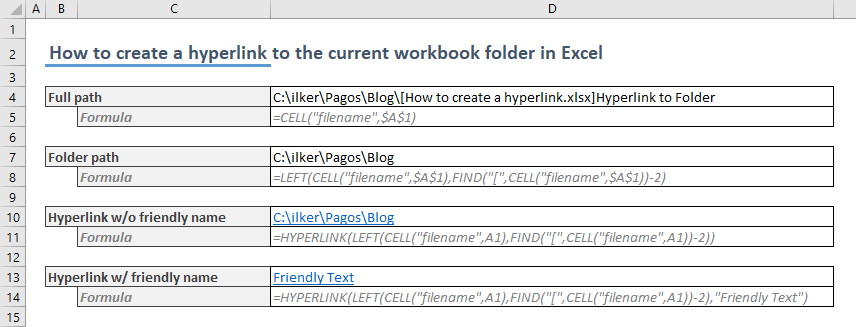## How to count the number of cells greater than the average in Excel

In this article, we are going to show you how to count the number of cells greater than the average in Excel. Download Workbook Formula =COUNTIFS(<numbers range>,”>“&AVERAGE(<numbers range>)) <numbers range>: The reference from the range of...## How to count the number of cells less than the average in Excel

In this article, we are going to show you how to count the number of cells that is less than the average of that data set in Excel. Download Workbook Formula =COUNTIFS(<numbers range>,”<“&AVERAGE(<numbers range>)) <numbers range>: The...## How to create a hyperlink to the current workbook folder in Excel

A hyperlink is not only for navigation between internet pages! Just like with websites, hyperlinks can be very useful in Excel too. You can even create hyperlinks to sheets or references in an Excel workbook as well. For example, you can generate a hyperlink to the...